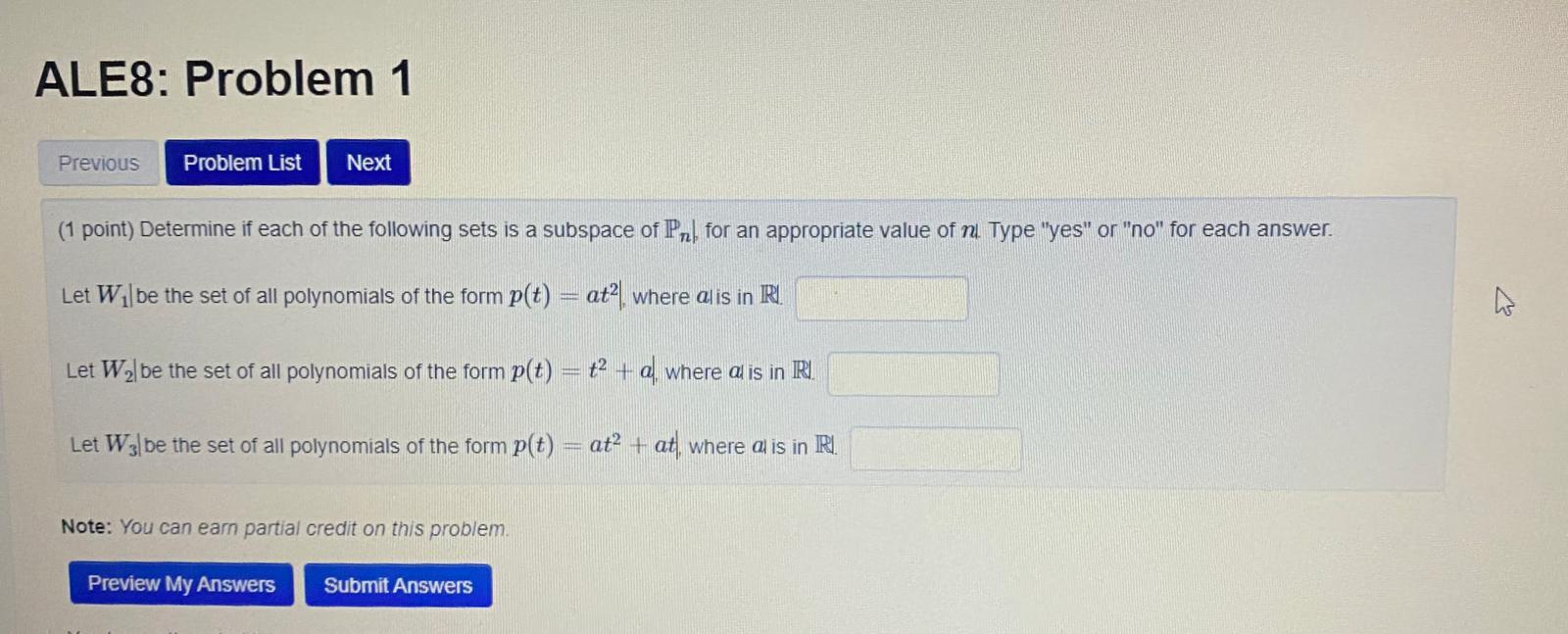### Create an Account

Already have account?

### Forgot Your Password ?

Home / Questions / ALES: Problem 1 Previous Problem List Next (1 point) Determine if each of the following se...

# ALES: Problem 1 Previous Problem List Next (1 point) Determine if each of the following sets is a subspace of Pr! for an appropriate value of nu Type "yes" or "no" for each answer. Let Wilbe the set o

ALES: Problem 1 Previous Problem List Next (1 point) Determine if each of the following sets is a subspace of Pr! for an appropriate value of nu Type "yes" or "no" for each answer. Let Wilbe the set of all polynomials of the form p(t) = atº, where alis in R. Let W2l be the set of all polynomials of the form p(t) = +2 + a where al is in R. Let W3]be the set of all polynomials of the form p(t) = at+ at, where al is in RI Note: You can earn partial credit on this problem. Preview My Answers Submit AnswersApr 14 2021 View more View Less

#### Answer (Solved)Subscribe To Get Solution